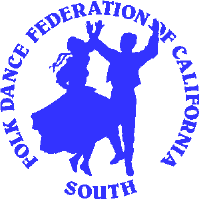Folk Dance Federation of California, South, Inc. Rhythms By Dick Oakes

Western music played for dances is usually notated in two, three, or four regular counts to a measure (on a musical score, the notes between two bars). Played against these even measures you also may find irregular rhythms (also called broken, uneven, or asymmetrical rhythms). For example, the rhythm pattern of a rhumba having a time signature of 4/4 (four quarter note counts to a measure) is with intervals of slow-quick-quick with the slow being held for two counts and the quicks being one count each.

In other parts of the world, other measures with complicated underlying rhythms are popular. A measure may have five, seven, nine, eleven, or thirteen counts, and some rhythms even have combinations of these measures. These irregular rhythms often are notated in slow and quick intervals, usually with a slow having a duration of three beats and a quick having two beats. To throw a hiccup into the mix, however, some intervals are slower (long) with four beats, or quicker (short) with one beat, or quickest (shortest) with one-half (.5) beat — the "&" count! And, on top of that, some dances are done to music of two or more rhythm patterns, such as Pravo Horo (Bulgarian) being danced to music with the assigned time signatures of 2, 4, or 6 counts!

 Time 7/8 One measure of seven eighth notes Counts 1 2 3 4 5 6 7 Rhythm Slow (S) Quick (Q) Quick (Q) Beats 3 2 2

Tempo, or the speed of a musical piece, adds another dimension. In the music to which the Greek Syrtaki is danced, two, three, and four distinct even tempos are known, which makes it hard to ascribe any one time signature to the parts of the music. For example, if the first slow tempo is notated with a rhythmic element of 4/4 (four quarter notes) and the third part, twice as fast, is notated as 2/4 (two quarter notes), what should be written for the tempo between these two? What should be written for the fourth tempo in a four-tempo piece?

To help us out of this dilemma, we can simply say that a melody is in "seven," for example, meaning that there are seven major even counts per measure, whatever the tempo or whatever the rhythm employed during those seven counts.

Here are a few examples of rhythms along with one or more dances with which they are associated.

 RHYTHMS 2 1 + 1 = 2 Pravo Horo (Bulgarian), Polka (Polish, international), 3 2 + 1 = 3 1 + 1 + 1 = 3 Tsamikos (Greek) Waltz (Slovenian), Hambo (Swedish) 4 2 + 2 = 4 2 + 1 + 1 = 4 1 + 1 + 1 + 1 = 4 Pravo Horo (Bulgarian), Pinosavka (Serbian) Syrtos (Greek), Misirlou (Greek/American) Vari Hasapiko (Greek) 5 2 + 3 = 5 Pajduško Horo (Bulgarian), Rustemul (Romanian) 6 3 + 3 = 6 Pravo Horo (Bulgarian) 7 3 + 4 = 7 3 + 1 + 3 3 + 2 + 2 = 7 2 + 2 + 3 = 7 2 + 1 + 2 + 2 = 7 2 + 2 + 1 + 2 = 7 Kostursko Oro (Greek Macedonian) Lesnoto Oro (Macedonian) Kalamatianos (Greek), Četvorno (Bulgarian) Rŭčenica (Bulgarian) Karamfil (Bulgarian) Eleno Mome (Bulgarian) 8 3 + 3 + 2 = 8 3 + 2 + 3 = 8 2 + 1 + 2 + 1 + 2 = 8 Čoček (Macedonian) Katerino Mome (Bulgarian) Învîrtita (Romanian) 9 2 + 3 + 2 + 2 = 9 2 + 2 + 2 + 3 = 9 2 + 2 + 2 + 3 = 9 2 + 2 + 2 + 1 + 2 = 9 Fatiše Kolo (Serbian) Niška Banja (Serbian), Karsilamas (Greek) Dajčovo Horo (Bulgarian) Tetovsko Oro (Macedonian) 10 3 + 2 + 2 + 3 = 10 Jurjuna (Armenian) 11 2 + 2 + 3 + 2 + 2 = 11 3 + 2 + 2 + 2 + 2 = 11 .5 + 1 + .5 + 1 + 1 + .5 + 1 + .5 + 1 + 1 + 1 = 11 (1       2        3    4     5       6         7    8    9)  (major beats) Kopanica (Gankino, Krivo) (Bulgarian) Ovčepolsko Oro (Macedonian) Zeybekiko (Ziembekiko) (Greek) 12 3 + 2 + 2 + 3 + 2 = 12 2 + 2 + 3 + 2 + 3 = 12 2 + 2 + 2 + 3 + 3 = 12 Beranče (Macedonian) Dolgoto (Macedonian) Topansko (Macedonian) 13 2 + 2 + 2 + 3 + 2 + 2 = 13 2 + 2 + 2 + 2 + 2 + 3 = 13 3 + 2 + 2 + 2 + 2 + 2 = 13 Postupano (Macedonian) Petrunino (Bulgarian) Elenino Horo (Bulgarian) 14 2 + 2 + 2 + 3 + 2 + 3 = 14 Krivo Sadovsko Horo (Bulgarian) 15 2 + 2 + 2 + 2 + 3 + 2 + 2 = 15 Bučimiš (Bulgarian) 21 (2 + 2 + 2 + 3 = 9) + (2 + 2 + 3 = 7) + (2 + 3 = 5) = 21 Zenkov (Russian) 22 (2 + 2 + 2 + 3 = 9) + (2 + 2 + 2 + 3 = 9) + (2 + 2 = 4) = 22 Sandansko Horo (Bulgarian) — dajčovo-dajčovo-pravo 25 (3 + 2 + 2 = 7) + (3 + 2 + 2 = 7) + (2 + 2 + 3 + 2 + 2 = 11) = 25 Sedi Donka (Bulgarian) — četvorno-četvorno-gankino